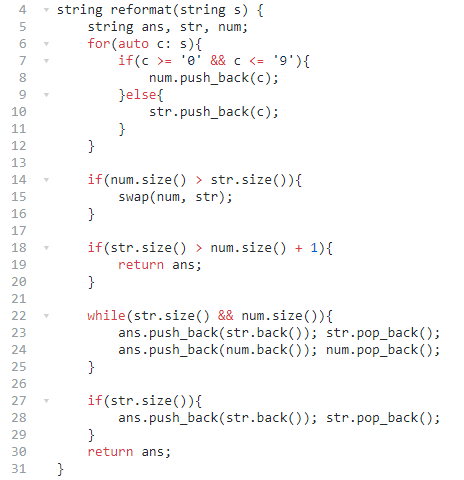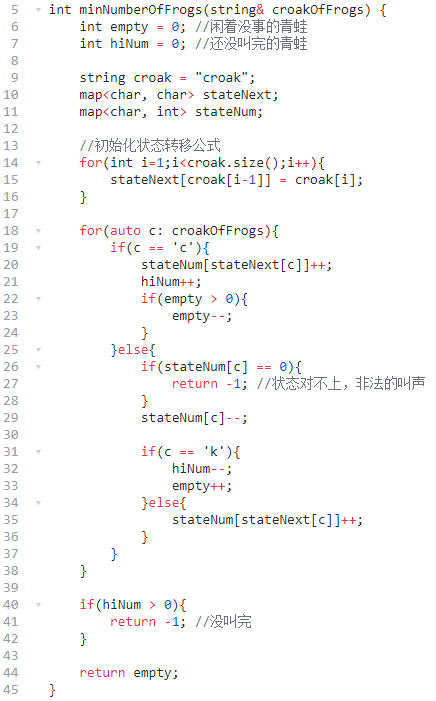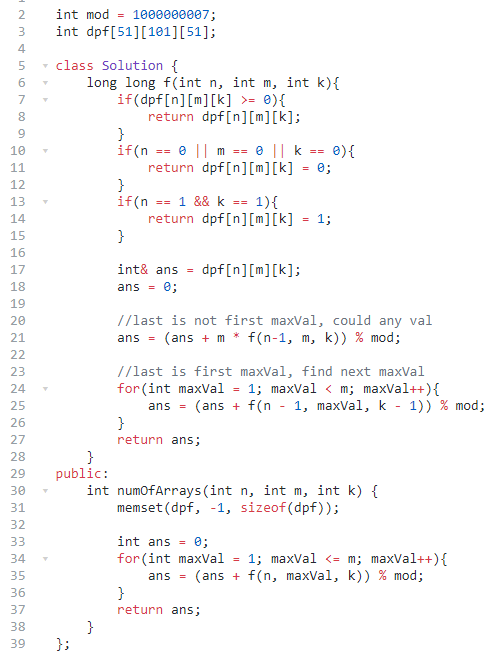# leetcode 第185算法比赛

## 一、重新格式化字符串## 二、点菜展示表

set 统计有哪些菜。
map 统计每个餐桌点了哪些菜。## 三、数青蛙## 四、生成数组

``````f(n,m,k)=sum(F(n,m,k,maxPos))
F(n,m,k,maxPos) = sum(maxVal^(n-maxPos) * f(maxPos-1,maxVal-1,k-1))
``````

``````f(n,m,k)=sum(F(n,maxVal,k))
F(n,m,k)=m*F(n-1,m,k)+sum(F(m-1,maxVal,k-1))
``````## 五、最后

《完》

-EOF-

QQ算法群：165531769（不止算法）

## 关注公众号,接收最新消息

1.## 关注小密圈,学习各种算法

1.